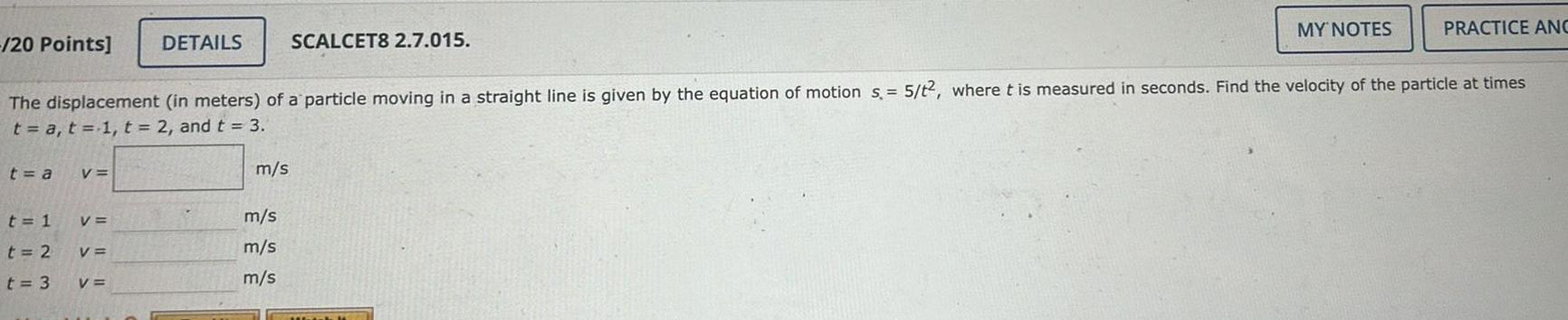Question:

# 20 Points t a t 1 t 2 t 3 V The displacement in meters of a

Last updated: 9/18/202320 Points t a t 1 t 2 t 3 V The displacement in meters of a particle moving in a straight line is given by the equation of motion s 5 t2 where t is measured in seconds Find the velocity of the particle at times t a t 1 t 2 and t 3 V V DETAILS V m s SCALCET8 2 7 015 m s m s m s MY NOTES PRACTICE ANC